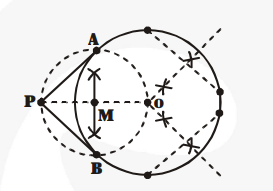# Draw a circle with the help of a bangle.Question:

Draw a circle with the help of a bangle. Take a point outside the circle. Construct the pair of tangents from this point to the circle.

Solution:

Steps of construction :1. Locate the centre O of the circle by drawing right bisectors of two non-parallel chords of the circle. These right bisectors intersect each other at the centre of the circle. (i.e., at O).

2. Take point P outside the circle and join OP.

3. Locate mid-point M of OP.

4. Taking M as centre and radius equal to MP, draw circle. It intersects the given circle at A and B.

5. Joint PA and PB.

Now, PA and PB are the required tangents drawn from P to the circle.